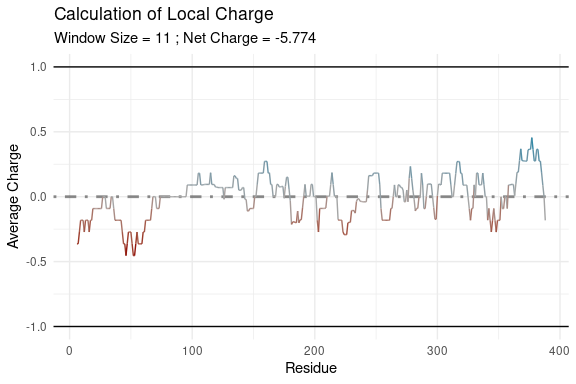# Charge and Hydropathy Vignette

library(idpr)

## Background

The composition of amino acids and the overall chemistry of Intrinsically Disordered Proteins (IDPs) are distinctly different from that of ordered proteins. Each amino acid has unique chemical characteristics that can either favor a compact or an extended structure (Uversky, 2019) . Disorder-promoting residues, those enriched in IDPs, are typically hydrophilic, charged, or small residues. Order promoting residues, those enriched in structured proteins, tend to be aliphatic, hydrophobic, aromatic, or form tertiary structures (Uversky, 2013).
Therefore, there is a distinct difference of biochemistry between IDPs and ordered proteins.

It was shown in Uversky, Gillespie, & Fink (2000) that both high net charge and low mean hydropathy are properties of IDPs. One explanation is that a high net charge leads to increased repulsion of residues causing an extended structure and low hydrophobicity will reduce the hydrophobic interactions causing reduced protein packing. When both average net charge and mean scaled hydropathy are plotted, IDPs occupy a unique area on the plot. The barrier between unfolded and compact proteins is: $<R> = 2.785 <H> - 1.151$ where <R> is the absolute mean net charge and <H> is the mean scaled hydropathy (Uversky, Gillespie, & Fink, 2000).

An alternative version of the Charge Hydropathy plot mentioned was shown in Uversky (2016), where the average net charge is shown rather than the absolute value. This creates two cutoff lines. One for positively charged peptides: $<R> = 2.785 <H> - 1.151$ and another for negatively charged peptides: $<R> = - 2.785 <H> + 1.151$ (Uversky, 2016).

This plot allows a distinction between negative and positive proteins while preserving the information of the charge-hydropathy plot.

Further, a this can be used to identify folded regions on a protein. FoldIndex used this equation and set variables to 0 and using a sliding window, the resulting values would identify regions predicted as folded or unfolded. $Score = 2.785 <H> - \lvert<R>\rvert -1.151$ When windows have a negative score (<0) sequences are predicted as disordered. When windows have a positive score (>0) sequences are predicted as ordered. This was described in Prilusky, J., Felder, C. E., et al. (2005).

## Installation

The idpr package can be installed from Bioconductor with the following line of code. It requires the BiocManager package to be installed

#BiocManager::install("idpr") 

The most recent version of the package can be installed with the following line of code. This requires the devtools package to be installed.

#devtools::install_github("wmm27/idpr")

## Methods

Methods are originally described in Uversky, Gillespie, & Fink (2000). The calculations shown here are to explain how chargeHydropathyPlot() obtains the values for graphing, and are done automatically within the function.

Mean scaled hydropathy is calculated by normalizing the Kyte and Doolittle scale on a scale of 0 to 1, with Arg having a hydropathy of 0 and Ile having a hydropathy of 1, averaged by the sequence length (Kyte & Doolittle, 1982; Uversky, 2016).

Net charge is calculated with the Henderson-Hasselbalch equation (Po & Senozan, 2001). While there is not one agreed upon set of pKa values, the Isoelectric Point Calculator does a great job at unifying these data sets (Kozlowski, 2016). The net charge is then averaged over the sequence length.

Both values are plotted in the Charge-Hydropathy plot to identify a protein or proteins location in that space.

### Example calulations

Examples will use the H. sapiens TP53 sequence, acquired from UniProt (UniProt Consortium 2019) and stored within the idpr package for examples.

HUMAN_P53 <- TP53Sequences
print(HUMAN_P53)
#>                                                                                                                                                                                                                                                                                                                                                                                            P04637|P53_HUMAN
#> "MEEPQSDPSVEPPLSQETFSDLWKLLPENNVLSPLPSQAMDDLMLSPDDIEQWFTEDPGPDEAPRMPEAAPPVAPAPAAPTPAAPAPAPSWPLSSSVPSQKTYQGSYGFRLGFLHSGTAKSVTCTYSPALNKMFCQLAKTCPVQLWVDSTPPPGTRVRAMAIYKQSQHMTEVVRRCPHHERCSDSDGLAPPQHLIRVEGNLRVEYLDDRNTFRHSVVVPYEPPEVGSDCTTIHYNYMCNSSCMGGMNRRPILTIITLEDSSGNLLGRNSFEVRVCACPGRDRRTEEENLRKKGEPHHELPPGSTKRALPNNTSSSPQPKKKPLDGEYFTLQIRGRERFEMFRELNEALELKDAQAGKEPGGSRAHSSHLKSKKGQSTSRHKKLMFKTEGPDSD"

The first portion is calculating the mean scaled hydropathy of the sequence. This will correspond to the value on the X-axis

P53_msh <- meanScaledHydropathy(HUMAN_P53)
print(P53_msh)
#>  0.4159389

The second value is the net charge. Setting averaged = TRUE will average the calculated net charge by the sequence length.This will correspond to the value on the Y-axis. Total Net charge can be calculated by setting averaged to FALSE.

P53_nc <- netCharge(HUMAN_P53,
averaged = TRUE)
print(P53_nc)
#>  -0.01469136

The chargeHydropathyFunction will automatically calculate these values and plot it as a point within the charge-hydropathy space according to Uversky, Gillespie, & Fink (2000) / Uversky (2016)

Since a ggplot is returned, the user can assign the plot to an object to modify aesthetics and/or add annotations. The example shown here will label the cooridnates on the returned plot.

chargeHydropathyPlot(HUMAN_P53) +
ggplot2::annotate("text",
x = P53_msh,
y = P53_nc + 0.1, #offset from point
label = paste("(", P53_msh, ", ", P53_nc, ")",
collapse = "",
sep = ""))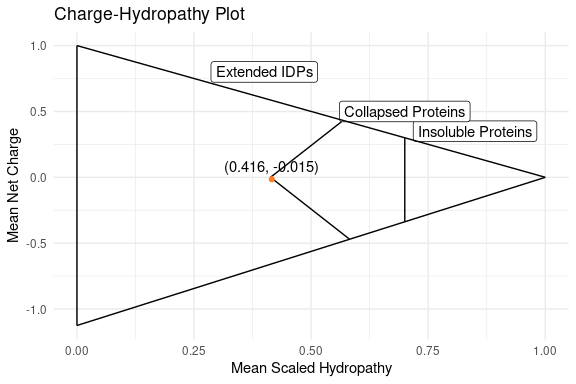## Using the chargeHydropathyPlot Function

The plot above shows calculations for one protein, but the function can accept multiple sequences.

The following examples will use highly similar TP53 sequences, acquired from UniProt (UniProt Consortium 2019) that were stored within the idpr package for examples.

TP53_Sequences <- TP53Sequences
print(TP53_Sequences)
#>                                                                                                                                                                                                                                                                                                                                                                                                      P02340|P53_MOUSE
#>              "MTAMEESQSDISLELPLSQETFSGLWKLLPPEDILPSPHCMDDLLLPQDVEEFFEGPSEALRVSGAPAAQDPVTETPGPVAPAPATPWPLSSFVPSQKTYQGNYGFHLGFLQSGTAKSVMCTYSPPLNKLFCQLAKTCPVQLWVSATPPAGSRVRAMAIYKKSQHMTEVVRRCPHHERCSDGDGLAPPQHLIRVEGNLYPEYLEDRQTFRHSVVVPYEPPEAGSEYTTIHYKYMCNSSCMGGMNRRPILTIITLEDSSGNLLGRDSFEVRVCACPGRDRRTEEENFRKKEVLCPELPPGSAKRALPTCTSASPPQKKKPLDGEYFTLKIRGRKRFEMFRELNEALELKDAHATEESGDSRAHSSYLKTKKGQSTSRHKKTMVKKVGPDSD"
#>                                                                                                                                                                                                                                                                                                                                                                                                      P04637|P53_HUMAN
#>           "MEEPQSDPSVEPPLSQETFSDLWKLLPENNVLSPLPSQAMDDLMLSPDDIEQWFTEDPGPDEAPRMPEAAPPVAPAPAAPTPAAPAPAPSWPLSSSVPSQKTYQGSYGFRLGFLHSGTAKSVTCTYSPALNKMFCQLAKTCPVQLWVDSTPPPGTRVRAMAIYKQSQHMTEVVRRCPHHERCSDSDGLAPPQHLIRVEGNLRVEYLDDRNTFRHSVVVPYEPPEVGSDCTTIHYNYMCNSSCMGGMNRRPILTIITLEDSSGNLLGRNSFEVRVCACPGRDRRTEEENLRKKGEPHHELPPGSTKRALPNNTSSSPQPKKKPLDGEYFTLQIRGRERFEMFRELNEALELKDAQAGKEPGGSRAHSSHLKSKKGQSTSRHKKLMFKTEGPDSD"
#>                                                                                                                                                                                                                                                                                                                                                                                                        P10361|P53_RAT
#>             "MEDSQSDMSIELPLSQETFSCLWKLLPPDDILPTTATGSPNSMEDLFLPQDVAELLEGPEEALQVSAPAAQEPGTEAPAPVAPASATPWPLSSSVPSQKTYQGNYGFHLGFLQSGTAKSVMCTYSISLNKLFCQLAKTCPVQLWVTSTPPPGTRVRAMAIYKKSQHMTEVVRRCPHHERCSDGDGLAPPQHLIRVEGNPYAEYLDDRQTFRHSVVVPYEPPEVGSDYTTIHYKYMCNSSCMGGMNRRPILTIITLEDSSGNLLGRDSFEVRVCACPGRDRRTEEENFRKKEEHCPELPPGSAKRALPTSTSSSPQQKKKPLDGEYFTLKIRGRERFEMFRELNEALELKDARAAEESGDSRAHSSYPKTKKGQSTSRHKKPMIKKVGPDSD"
#>                                                                                                                                                                                                                                                                                                                                                                                                      Q29537|P53_CANLF
#>                       "MEESQSELNIDPPLSQETFSELWNLLPENNVLSSELCPAVDELLLPESVVNWLDEDSDDAPRMPATSAPTAPGPAPSWPLSSSVPSPKTYPGTYGFRLGFLHSGTAKSVTWTYSPLLNKLFCQLAKTCPVQLWVSSPPPPNTCVRAMAIYKKSEFVTEVVRRCPHHERCSDSSDGLAPPQHLIRVEGNLRAKYLDDRNTFRHSVVVPYEPPEVGSDYTTIHYNYMCNSSCMGGMNRRPILTIITLEDSSGNVLGRNSFEVRVCACPGRDRRTEEENFHKKGEPCPEPPPGSTKRALPPSTSSSPPQKKKPLDGEYFTLQIRGRERYEMFRNLNEALELKDAQSGKEPGGSRAHSSHLKAKKGQSTSRHKKLMFKREGLDSD"
#>                                                                                                                                                                                                                                                                                                                                                                                                      Q00366|P53_MESAU
#>                                                                                                                                                                                                                                                                                                                                                                                                      O09185|P53_CRIGR
#>                                                                                                                                                                                                                                                                                                                                                                                                      Q9TTA1|P53_TUPBE
#>           "MEEPQSDPSVEPPLSQETFSDLWKLLPENNVLSPLPSQAMDDLMLSPDDIEQWFTEDPGPDEAPRMPEAAPPVAPAPAAPTPAAPAPAPSWPLSSSVPSQKTYQGSYGFRLGFLHSGTAKSVTCTYSPDLNKLFCQLAKTCPVQLWVDSAPPPGTRVRAMAIYKQSQYVTEVVRRCPHHERCSDSDGLAPPQHLIRVEGNLHAEYSDDRNTFRHSVVVPYEPPEVGSDCTTIHYNYMCNSSCMGGMNRRPILTIITLEDSSGKLLGRNSFEVRICACPGRDRRTEEENFRKKGESCPKLPTGSIKRALPTGSSSSPQPKKKPLDEEYFTLQIRGRERFEMLREINEALELKDAMAGKESAGSRAHSSHLKSKKGQSTSRHRKLMFKTEGPDSD"
#>                                                                                                                                                                                                                                                                                                                                                                                                      Q95330|P53_RABIT
#>             "MEESQSDLSLEPPLSQETFSDLWKLLPENNLLTTSLNPPVDDLLSAEDVANWLNEDPEEGLRVPAAPAPEAPAPAAPALAAPAPATSWPLSSSVPSQKTYHGNYGFRLGFLHSGTAKSVTCTYSPCLNKLFCQLAKTCPVQLWVDSTPPPGTRVRAMAIYKKSQHMTEVVRRCPHHERCSDSDGLAPPQHLIRVEGNLRAEYLDDRNTFRHSVVVPYEPPEVGSDCTTIHYNYMCNSSCMGGMNRRPILTIITLEDSSGNLLGRNSFEVRVCACPGRDRRTEEENFRKKGEPCPELPPGSSKRALPTTTTDSSPQTKKKPLDGEYFILKIRGRERFEMFRELNEALELKDAQAEKEPGGSRAHSSYLKAKKGQSTSRHKKPMFKREGPDSD"
#>                                                                                                                                                                                                                                                                                                                                                                                           A0A2I2Y7Z8|A0A2I2Y7Z8_GORGO
#> "MDDLMLSPDDIEQWFTEDPGPDEAPRMPEAAPPVAPAPAAPTPAAPAPAPSWPLSSSVPSQKTYQGSYGFRLGFLHSGTAKSVTCTYSPTLNKMFCQLAKTCPVQLWVDSTPPPGTRVRAMAIYKQSQHMTEVVRRCPHHERCSDSDGLAPPQHLIRVEGNLRVEYLDDRNTFRHSVVVPYEPPEVGSDCTTIHYNYMCNSSCMGGMNRRPILTIITLEDSSGNLLGRNSFEVRVCACPGRDRRTEEENLRKKGEPHHELPPGSTKRALPNNTSSSPQPKKKPLDGEYFTLQIRGRERFEMFRELNEALELKDAQAGKEPGGRNAKHSPGDPDPPLSETFNLNICPYPAGKLELLKLSPCPCLCRQVTLMSFLFFLIFFYFRLYWGIIEPPKLHTFKVCSVMI"
gg <- chargeHydropathyPlot(
sequence = TP53_Sequences,
pKaSet = "IPC_protein")
plot(gg)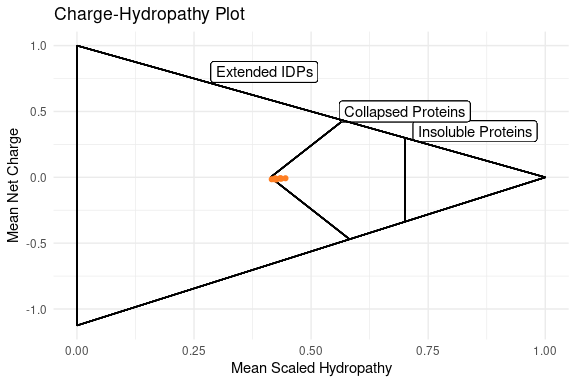Since it is a ggplot, the user can add additional parameters to the plot like labels, custom themes, and other supported features. An example of this is shown in “Methods”.

If you do not wish to use the IPC_protein pKa set, you can change this to any accepted by netCharge().

chargeHydropathyPlot(
sequence = TP53_Sequences,
pKaSet = "EMBOSS")  #Using EMBOSS pKa set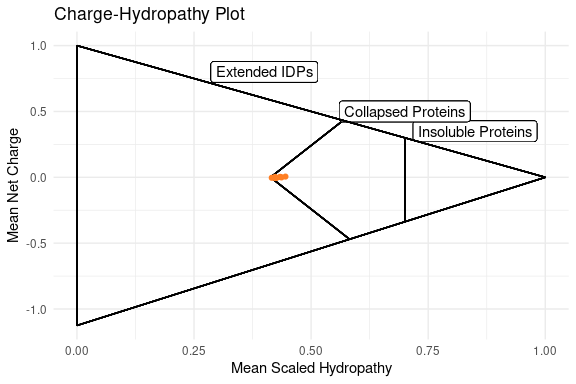## Using FoldIndexR to predict folded and unfolded windows.

foldIndexR(sequence = HUMAN_P53,
plotResults = TRUE)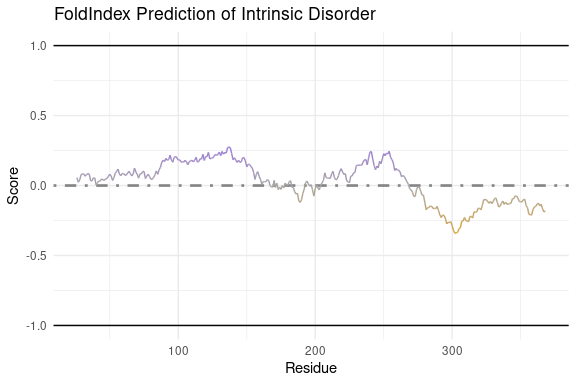Prilusky, J., Felder, C. E., et al. (2005).

## Calculating Scaled Hydropathy

### Mean Scaled Hydropathy

meanScaledHydropathy is used to calculate the scaled hydropathy of a sequence. See the introduction and methods for more details.

 meanScaledHydropathy(HUMAN_P53)
#>  0.4159389

### Global Hydropathy

scaledHydropathyGlobal is used to match the scaled hydropathy of an amino acid sequence for each residue in the sequence.

The results can be a data frame of matched values. It will result in a data frame with 3 columns. The first column is “Position”, which indicates the numeric position of the residue in the submitted sequence. The second column is “AA”, which indicates the amino acid residue as a single letter. The third column is “Hydropathy”, which indicates the value of scaled hydropathy for that residue, which was matched by the function.

P53_shg <- scaledHydropathyGlobal(HUMAN_P53)
#>   Position AA Hydropathy
#> 1        1  M      0.711
#> 2        2  E      0.111
#> 3        3  E      0.111
#> 4        4  P      0.322
#> 5        5  Q      0.111
#> 6        6  S      0.411

Or results can be returned as a plot

scaledHydropathyGlobal(HUMAN_P53,
plotResults = TRUE)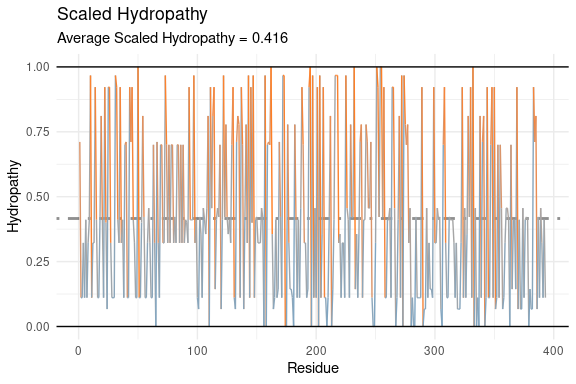(This is not the most aesthetically pleasing plot, so a sequenceMap from idpr is reccomended in this case for visualizations.)

P53_shg <- scaledHydropathyGlobal(HUMAN_P53)
sequenceMap(sequence = P53_shg$AA, property = P53_shg$Hydropathy,
customColors = c("chocolate1", "grey65", "skyblue3"))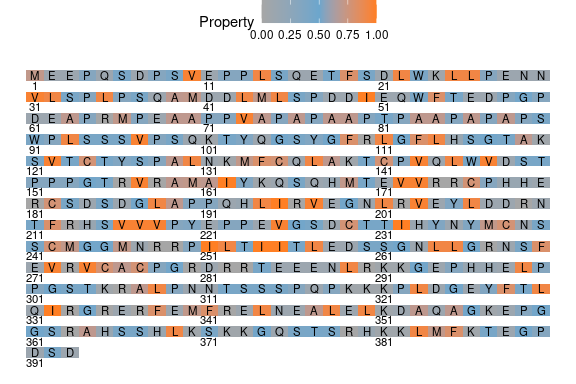### Local Hydrophobicity

scaledHydropathyLocal is a function used to calculate the average hydrophobicity of a sequence using a sliding window. The results are returned as a data frame or a plot.

The scaled hydropathy is matched to each residue then the average of each window is calculated.

This can be helpful to identify regions of high or low hydrophobicity within a protein. This may help with identifying IDRs as well.

Results can be returned as a data frame of window hydropathy scores. It will result in a data frame with 3 columns. The first column is “Position”, which indicates the numeric position of the residue in the submitted sequence. The second column is “Window” which indicates all the residues within the sliding window that were used for calculations. The “CenterResidue” column specifies the amino acid residue as a single letter that is located in the center of the window and is located at the number specified by “Position” And finally, the “WindowHydropathy” is the calculated average hydropathy for the residues specified in the “Window” column.

P53_shl <- scaledHydropathyLocal(HUMAN_P53,
plotResults = FALSE)
#>   Position    Window CenterResidue WindowHydropathy
#> 1        5 MEEPQSDPS             Q        0.2912222
#> 2        6 EEPQSDPSV             S        0.3196667
#> 3        7 EPQSDPSVE             D        0.3196667
#> 4        8 PQSDPSVEP             P        0.3431111
#> 5        9 QSDPSVEPP             S        0.3431111
#> 6       10 SDPSVEPPL             V        0.4332222

The results can also return a plot that shows each window’s hydropathy score along the sequence.

scaledHydropathyLocal(HUMAN_P53,
plotResults = TRUE)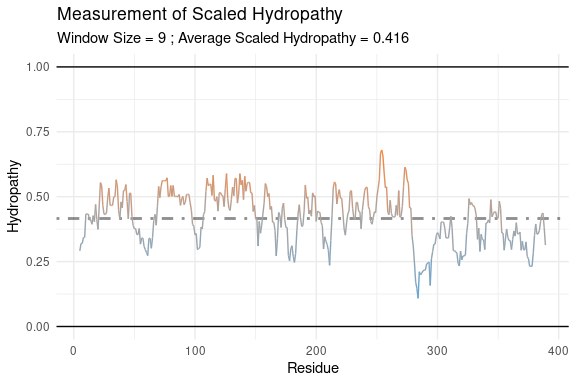The window size can be specified as well by the “window” argument. This must be an odd integer

scaledHydropathyLocal(HUMAN_P53,
window = 3,
plotResults = TRUE)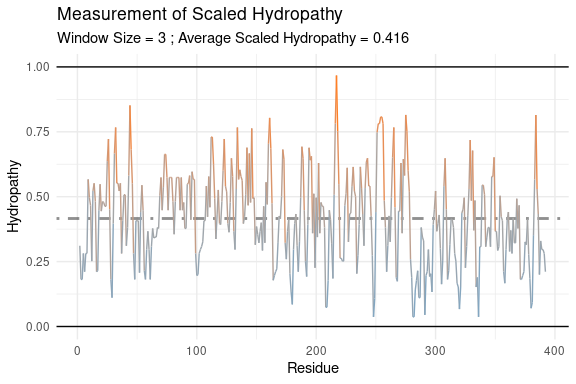## Calculating Charge

### Net Charge

netCharge is used to calculate the net charge of a sequence. See the introduction and methods for more details.

netCharge(HUMAN_P53)
#>  -5.773703

Setting averaged = TRUE will average the calculated net charge by the sequence length (Shown before in methods).

netCharge(HUMAN_P53,
averaged = TRUE)
#>  -0.01469136

netCharge relies on the Henderson-Hasselbalch equation (via the hendersonHasselbalch function). Therefore the pH and pKa are critical for calculations. netCharge allows for different pH values using the pH argument.

netCharge(HUMAN_P53,
pH = 5.5)
#>  5.572653
netCharge(HUMAN_P53,
pH = 7)
#>  -5.773703
netCharge(HUMAN_P53,
pH = 8)
#>  -13.01845

There are also many pKa sets that are preloaded in idpr. pKa datasets used within this vignette are cited. See the documentation for netCharge or pKaData within idpr for additional information and citations for available pKa sets. Additionally, see Kozlowski (2016) for further details on pKa data sets.

• “EMBOSS” - (Rice, Longden, & Bleasby, 2000)
• “DTASelect”
• “Solomons”
• “Sillero”
• “Rodwell”
• “Lehninger”
• “Toseland”
• “Thurlkill”
• “Nozaki”
• “Dawson”
• “Bjellqvist”
• “ProMoST”
• “Vollhardt”
• “IPC_protein” (Kozlowski, 2016).
• “IPC_peptide” (Kozlowski, 2016).
netCharge(HUMAN_P53,
pKaSet = "IPC_protein")
#>  -5.773703
netCharge(HUMAN_P53,
pKaSet = "IPC_peptide")
#>  -3.286671
netCharge(HUMAN_P53,
pKaSet = "EMBOSS")
#>  -1.404403

Alternatively, the user may supply a custom pKa dataset. The format must be a data frame where: Column 1 must be a character vector of residues AND Column 2 must be a numeric vector of pKa values. This can be helpful if there is a data set the user prefers or if there are noncannonical amino acids. Here is an example using the pKa values from Wikipedia (Proteinogenic amino acid, n.d.). https://en.wikipedia.org/wiki/Proteinogenic_amino_acid#Chemical_properties

print(custom_pKa)
#>    AA   pKa
#> 1   C  8.55
#> 2   D  3.67
#> 3   E  4.25
#> 4   H  6.54
#> 5   K 10.40
#> 6   R 12.30
#> 7   Y  9.84
#> 8 NH3  9.28
#> 9 COO  1.99

netCharge(HUMAN_P53,
pKaSet = custom_pKa,
includeTermini = FALSE)
#>  -1.143015

### Global Charge Distibution

chargeCalculationGlobal is a function used to calculate the charge of each residue, independent of other amino acids, within a sequence. The results are returned as a data frame (default) or a plot.

chargeCalculationGlobal accepts the same pKa and pH arguments as netCharge.

The results can return a data frame of charge for each residue

It will result in a data frame with 3 columns. The first column is “Position”, which indicates the numeric position of the residue in the submitted sequence. The second column is “AA”, which indicates the amino acid residue as a single letter. The third column is “Charge”, which indicates the calculated charge for the residue at the specified pH.

P53_ccg <- chargeCalculationGlobal(HUMAN_P53)
#>   Position AA     Charge
#> 1        1  M  0.9920106
#> 2        2  E -0.9974244
#> 3        3  E -0.9974244
#> 4        4  P  0.0000000
#> 5        5  Q  0.0000000
#> 6        6  S  0.0000000

The results can return a ggplot visualizing the charge distribution.

chargeCalculationGlobal(HUMAN_P53,
plotResults = TRUE)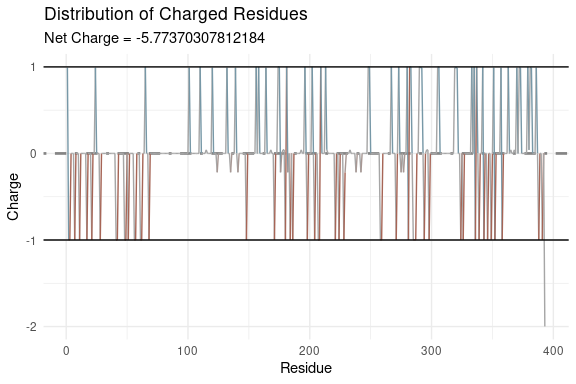(This is not the most aesthetically pleasing plot, so a sequenceMap from idpr is recommended in this case for visualizations.)

P53_ccg <- chargeCalculationGlobal(HUMAN_P53) #repeating from above
sequenceMap(sequence = P53_ccg$AA, property = P53_ccg$Charge,
customColors = c("red", "blue", "grey65"))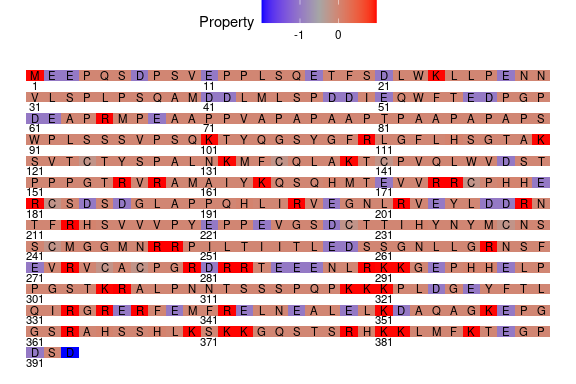The C-terminus here has a charge of ~ -2 since the function aggregates the termini values with residue charges by default. If you wish to calculate the termini as separate values, use sumTermini = FALSE. This will add 2 residues to the data frame as “NH3” and “COO”

P53_ccg <- chargeCalculationGlobal(HUMAN_P53,
sumTermini = FALSE)
#>   Position  AA     Charge
#> 1        0 NH3  0.9920106
#> 2        1   M  0.0000000
#> 3        2   E -0.9974244
#> 4        3   E -0.9974244
#> 5        4   P  0.0000000
#> 6        5   Q  0.0000000

If you wish to completely ignore the termini for calculation, set includeTermini = FALSE.

P53_ccg <- chargeCalculationGlobal(HUMAN_P53,
includeTermini = FALSE)
#>   Position AA     Charge
#> 1        1  M  0.0000000
#> 2        2  E -0.9974244
#> 3        3  E -0.9974244
#> 4        4  P  0.0000000
#> 5        5  Q  0.0000000
#> 6        6  S  0.0000000

### Local Charge

chargeCalculationLocal is a function used to calculate the charge of a sequence using a sliding window. The results are returned as a data frame (default) or a plot.

chargeCalculationLocal accepts the same pKa and pH arguments as netCharge.

Unlike chargeCalculationGlobal, the chargeCalculationLocal function does not consider termini for calculations.

Results can be returned as a data frame of window hydropathy scores. It will result in a data frame with 4 columns. The first column is “Position”, which indicates the numeric position of the residue in the submitted sequence. The “CenterResidue” column specifies the amino acid residue as a single letter that is located in the center of the window and is located at the number specified by “Position”. The “Window” column contains all the residues within the sliding window that were used for calculating the charge. And finally, the “windowCharge” is the calculated average charge for the residues specified in the “Window” column.

P53_cgl <- chargeCalculationLocal(HUMAN_P53)
#>   Position CenterResidue    Window windowCharge
#> 1        5             Q MEEPQSDPS   -0.3326783
#> 2        6             S EEPQSDPSV   -0.3326783
#> 3        7             D EPQSDPSVE   -0.3326783
#> 4        8             P PQSDPSVEP   -0.2218534
#> 5        9             S QSDPSVEPP   -0.2218534
#> 6       10             V SDPSVEPPL   -0.2218534

Alternatively, results can be returned as a plot of each window’s charge.

chargeCalculationLocal(HUMAN_P53,
plotResults = TRUE)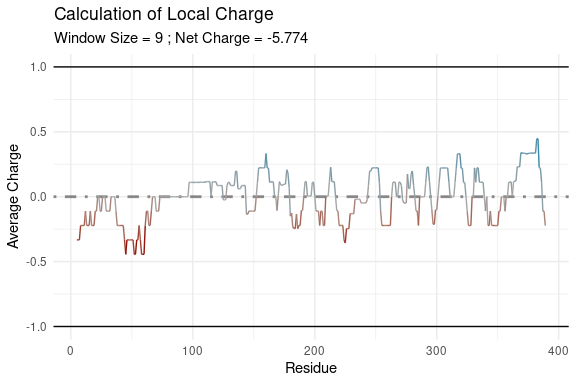The window size can be specified as well via the “window” argument. This must be an odd integer

chargeCalculationLocal(HUMAN_P53,
window = 11,
plotResults = TRUE)